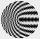# Sum of two primes

Christian Goldbach, a mathematician, found out that every even number greater than 2 can be expressed as a sum of two prime numbers. Write or express 2018 as a sum of two prime numbers.

Result

x = (Correct answer is: 1009 + 1009)#### Solution:Leave us a comment of example and its solution (i.e. if it is still somewhat unclear...):

Showing 0 comments:Be the first to comment!## Next similar examples:

1. Chebyshev formulaTo estimate the number of primes less than x is used Chebyshev formula: ? Estimate the number of primes less than 30300537.
2. SequenceBetween numbers 1 and 53 insert n members of the arithmetic sequence that its sum is 702.
3. SeatsSeats in the sport hall are organized so that each subsequent row has five more seats. First has 10 seats. How many seats are: a) in the eighth row b) in the eighteenth row
4. TicketsTickets to the zoo cost \$4 for children, \$5 for teenagers and \$6 for adults. In the high season, 1200 people come to the zoo every day. On a certain day, the total revenue at the zoo was \$5300. For every 3 teenagers, 8 children went to the zoo. How many te
5. Theorem proveWe want to prove the sentense: If the natural number n is divisible by six, then n is divisible by three. From what assumption we started?
6. Sheep and cowsThere are only sheep and cows on the farm. Sheep is eight more than cows. The number of cows is half the number of sheep. How many animals live on the farm?
7. Complex number coordinatesWhich coordinates show the location of -2+3i
8. Fish tankA fish tank at a pet store has 8 zebra fish. In how many different ways can George choose 2 zebra fish to buy?
9. Roman numeralsWrite numbers written in Roman numerals as decimal.
10. Geometric progression 2There is geometric sequence with a1=5.7 and quotient q=-2.5. Calculate a17.
11. Linsys2Solve two equations with two unknowns: 400x+120y=147.2 350x+200y=144
12. EquationEquation ? has one root x1 = 8. Determine the coefficient b and the second root x2.
13. AS sequenceIn an arithmetic sequence is given the difference d = -3 and a71 = 455. a) Determine the value of a62 b) Determine the sum of 71 members.
14. 75th percentile (quartille Q3)Find 75th percentile for 30,42,42,46,46,46,50,50,54
15. Sequence 3Write the first 5 members of an arithmetic sequence: a4=-35, a11=-105.
16. DiscriminantDetermine the discriminant of the equation: ?
17. Six termsFind the first six terms of the sequence a1 = -3, an = 2 * an-1